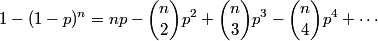# Probability mistake can give a good approximation

If you run into someone on the street, the probability that the other person shares your birthday is 1/365. If you run into five people, the probability that at least one of them shares your birthday is 5/365, right?

The answer 5/365 is quite accurate, but not exactly correct. It’s good enough for this problem since there are other practical difficulties besides the quality of the approximation. For example, the problem implicitly assumes there are 365 days in a year, i.e. that no one is ever born on Leap Day.

Now think about a similar problem. Suppose the chance of rain is 40% each day for the next three days. Does that mean there is a 120% chance that it will rain at least one of the next three days? That can’t be. In fact, the chance of some rain over the next three days is 78.4% (assuming the probabilities are independent, which they’re probably not).

The following rule appears to be correct for birthdays but not for predicting rain.

If the probability of a success in one attempt is p, then the probability of at least one success in n attempts is np.

Why does this rule hold sometimes and not at other times? If the probability of success on each attempt is p, the probability of failure on each attempt is (1-p). The probability of n failures in a row is (1-p)n and so the probability of at least one success is 1 – (1-p)n. That’s the right way to approach the birthday example and the rain example. In the birthday example, p = 1/365 and so the probability of running into at least one person in five who shares your birthday is 1 – (364/365)5 = 0.013624. And 5/365 = 0.013699 is a very good approximation. In the rain example, p = 0.4 and the probability of at least one day of rain out of the next three is 1 – 0.63 = 0.784. The difference between the birthday example and the rain example is the size of p. The following equation, based on the binomial theorem, explains why.When we use np as our approximation, we’re ignoring the terms involving p2 and higher powers of p. When p is small, higher powers of p are very small and can be ignored. That’s why the approximation worked well for p = 1/365. But when p is large, say p = 0.4, the error is large; the terms involving higher powers of p are important in that case. Notice also that the size of n matters. The birthday example breaks down when n is large. If you run into 400 people, it is likely that one of them will share your birthday, but far from certain. The probability in that case is about 2/3, not 400/365.

When p and n are both small, the probability of at least one success out of n tries is approximately np. We can say more. Because the first term the approximation drops from the equation above has a negative sign, our approximation is also an upper bound. This says np slightly over-estimates the probability.

Now how small do p and n have to be? If you calculate the approximation np and get a small answer, then it’s a good answer. Why? The error in the np approximation is roughly n(n-1)p2/2, which is less than (np)2. And if np is small, (np)2 is very small.

See Sales tax included for a similar discussion. That also post looks at a common mistake and explains why it makes a good approximation under some circumstances and not under others.

## 4 thoughts on “Probability mistake can give a good approximation”

1. Danny Tarlow

I guess it’s somewhat off-topic, but the thing that always bothers me about using the probability of rain in a given day as an example is the implicit assumption that rain on each day is an independent event. In reality most times, it’s more like there’s a 40% chance a storm will take a certain path through the region, and if the storm does take this path, there’s a near certain chance that it will rain for three days straight.

2. Good point. Assuming independence in weather reports is unrealistic. Birthdays, sure: independence seems justified, unless you run into twins walking together. :-)

3. teknas

The birthday paradox is a more interesting variant of the problem you introduce. Here you end up picking a pair of people in a room at random and calculate the probability of finding at least a pair of them who share their birthday. Turns out with 23 people you have 0.5 probability of a pair of them sharing their birthday. Not very intuitive but the math is simple and very insightful.

4. thank you! this was very concise and well explained. super helpful for my statistics class.